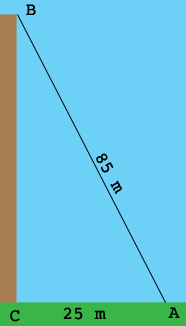SEARCH HOMEMath Central Quandaries & QueriesQuestion from Hayley: A young man is 36 meters away from a building and looking at a bird sitting on top of the building. The man knows he is 85 meters away from the bird. How tall is the building?Hi Hayley,

Draw a diagram!If the building is perpendicular to the ground then the angle $BCA$ is a right angle. Can you see how to calculate the length of the side $BC?$ Maybe Pythagoras can help.

PennyMath Central is supported by the University of Regina and The Pacific Institute for the Mathematical Sciences.